Related Articles

# How to change color of regression line in R ?

• Last Updated : 24 Jun, 2021

A regression line is basically used in statistical models which help to estimate the relationship between a dependent variable and at least one independent variable. In this article, we are going to see how to plot a regression line using ggplot2 in R programming language and different methods to change the color using a built-in data set as an example.

Dataset Used: Here we are using a built-in data frame “Orange” which consists of details about the growth of five different types of orange trees. The data frame has 35 rows and 3 columns. The columns in this data frame are :

• Tree: The ordering of trees on which experiment is made on the basis of increasing diameter values of the orange.
• Age: The age of the trees since when they were planted.
• Circumference: The circumference of the orange.

First, we will draw a scatter plot. We will use the function geom_point( ) to plot the scatter plot which comes under the ggplot2 library.

Syntax:

geom_point( mapping=NULL, data=NULL, stat=identity, position=”identity”)

Basically, we are doing a comparative analysis of the circumference vs age of the oranges. The function used is geom_smooth( ) to plot a smooth line or regression line.

Syntax:

geom_smooth(method=”auto”,se=FALSE,fullrange=TRUE,level=0.95)

Parameter :

• method : The smoothing method is assigned using the keyword loess, lm, glm etc
• lm : linear model, loess : default for smooth lines during small data set observations.
• formula : You can also use formulas for smooth lines. For example : y~poly(x,4) which will plot a smooth line of degree 4. Higher the degree more bends the smooth line will have.
• se : It takes logical values either “TRUE” or “FALSE”.
• fullrange : It takes logical value either “TRUE” or “FALSE”.
• level : By default level is 0.95 for the confidence interval.

Let us first draw a regular plot so that the difference is apparent.

Example:

## R

 `# Scatter Plot and Regression Line``library``(ggplot2)`` ` `ggplt <- ``ggplot``(Orange,``aes``(x=circumference,y=age))+``         ``geom_point``()+``         ``geom_smooth``(method=lm,se=``FALSE``,fullrange=``TRUE``)+``         ``theme_classic``()``ggplt`

Output: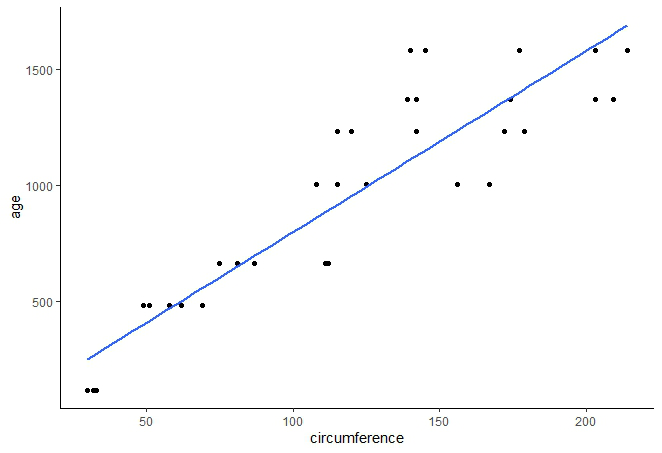By default, the regression line is blue. To change the color we have to use the keyword color inside the geom_smooth( ) function.

Syntax:

geom_smooth(method=”auto”, color=”color_name”)

Color code is of the form “#RedRedBlueBlueGreenGreen”

Example:

## R

 `# Change Regression Line Color using name of color``library``(ggplot2)`` ` `ggplt <- ``ggplot``(Orange,``aes``(x=circumference,y=age))+``         ``geom_point``()+``         ``geom_smooth``(method=lm,se=``FALSE``,fullrange=``TRUE``,color=``"cyan"``)+``         ``theme_classic``()``ggplt`

Output: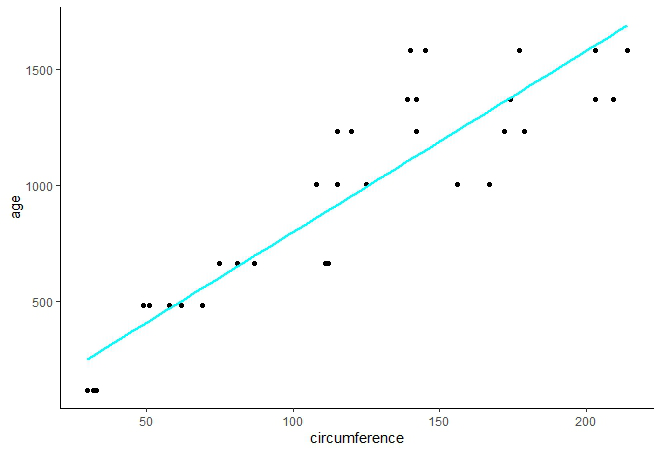Example:

## R

 `# Change Regression Line Color using Color Code``library``(ggplot2)`` ` `ggplt <- ``ggplot``(Orange,``aes``(x=circumference,y=age))+``         ``geom_point``()+``         ``geom_smooth``(method=lm,se=``FALSE``,fullrange=``TRUE``,color=``"#006000"``)+``         ``theme_classic``()``ggplt`

Output: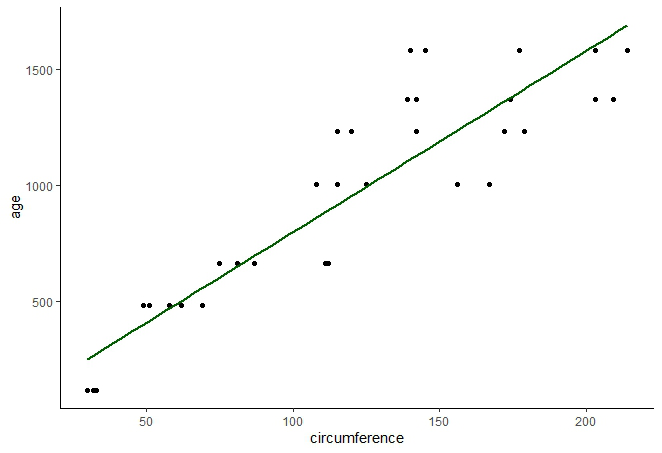### Multiple Regression Lines

The data frame consists of information about five different types of orange trees. So, let’s segregate the scatter points on the basis of the group “Tree”.  The shape of the points will be on the basis of the category of the trees.

Example:

## R

 `# Scatter Plot with multiple groups``library``(ggplot2)`` ` `ggplt <- ``ggplot``(Orange,``aes``(x=circumference,y=age,shape=Tree))+``         ``geom_point``()+``         ``theme_classic``()`` ` `ggplt`` ` `# Plotting Regression Lines on the basis``# of groups``ggplt+``geom_smooth``(method=lm,se=``FALSE``,fullrange=``TRUE``)`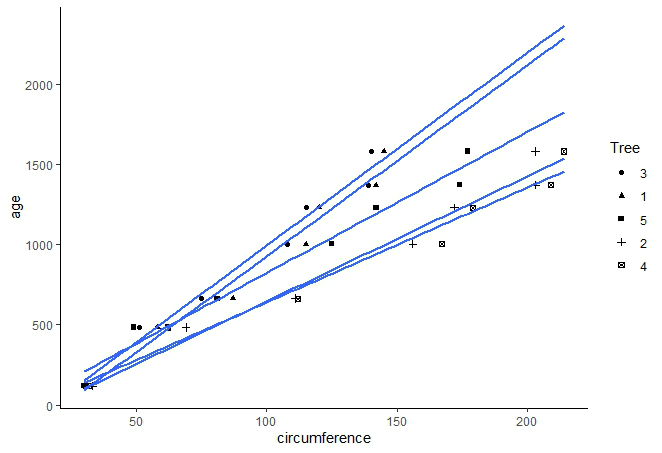We can see that all the regression lines are of the same color. Now we will see two different methods on how to assign different colors to multiple regression lines.

Method 1: Using color

It is the default method where color is assigned automatically by the R compiler. The key idea is to assign color on the basis of Trees since every Tree group has different regression lines. So, we write the below command inside the geom_smooth( ) function:

aes(color=grp_var)

grp_var : The column in the data frame through which grouping is done.

Example:

## R

 `# Scatter Plot``library``(ggplot2)`` ` `ggplt <- ``ggplot``(Orange,``aes``(x=circumference,y=age,shape=Tree))+``         ``geom_point``()+``         ``theme_classic``()``ggplt`` ` `# Plotting Regression Lines on the basis of groups``ggplt+``geom_smooth``(method=lm,se=``FALSE``,fullrange=``TRUE``)`` ` `# Changing color of Regression Lines manually``ggplt+``geom_smooth``(method=lm,se=``FALSE``,fullrange=``TRUE``,``aes``(color=Tree))`

Output: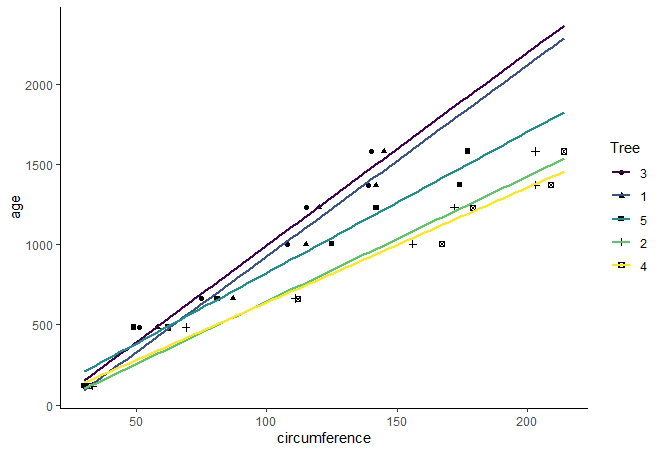Method 2: Changing Color Manually

1. Scale_color_manual( ): This function is used to add color manually on the basis of users’ choice.

Syntax:

scale_color_manual(values=c(“color1”, “color2” , “color3”,….))

color : color name or color code

Example:

## R

 `# Scatter Plot and Regression Line``library``(ggplot2)`` ` `ggplt <- ``ggplot``(Orange,``aes``(x=circumference,y=age,shape=Tree,color=Tree))+``         ``geom_point``()+``         ``geom_smooth``(method=lm,se=``FALSE``,fullrange=``TRUE``)+``         ``theme_classic``()`` ` `ggplt`` ` `# Manually changing color of Regression Lines ``ggplt+``scale_color_manual``(values=``c``(``"Red"``,``"Purple"``,``"#006000"``,``"Brown"``,``"Cyan"``))`

Output: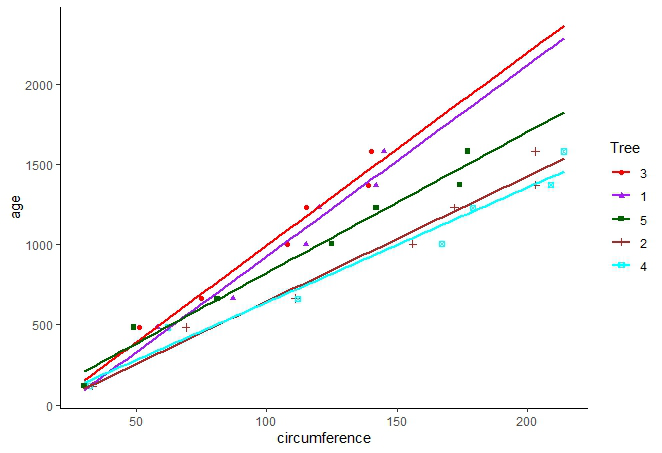2. Scale_color_brewer( ) : R provides us with various color palettes which contain different shades of color.

Syntax:

scale_color_brewer(palette=”palette_name”)

palette_name : The name of the palette. In our case we have used the palette “Greens”.

Example:

## R

 `# Scatter Plot and Regression Line``library``(ggplot2)`` ` `ggplt <- ``ggplot``(Orange,``aes``(x=circumference,y=age,shape=Tree,color=Tree))+``         ``geom_point``()+``         ``geom_smooth``(method=lm,se=``FALSE``,fullrange=``TRUE``)+``         ``theme_classic``()`` ` `ggplt`` ` `# Manually changing color of Regression Lines ``ggplt+``scale_color_brewer``(palette=``"Greens"``)`

Output: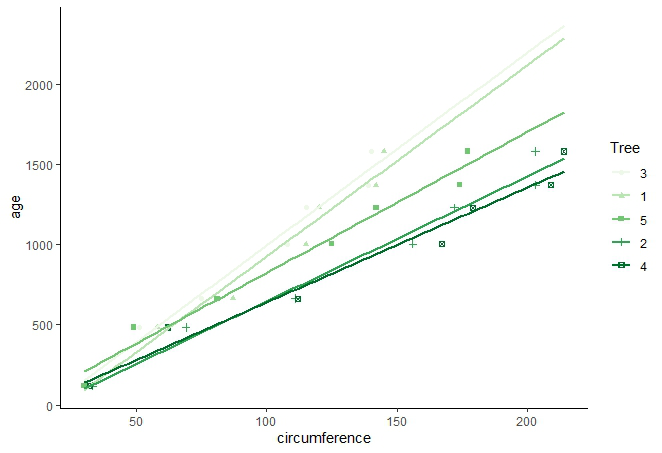3. Scale_color_grey( ): It is used to assign grayscale value to the regression lines. Simply invoke the function to add grayscale in regression lines.

Example:

## R

 `# Scatter Plot and Regression Line``library``(ggplot2)`` ` `ggplt <- ``ggplot``(Orange,``aes``(x=circumference,y=age,shape=Tree,color=Tree))+``         ``geom_point``()+``         ``geom_smooth``(method=lm,se=``FALSE``,fullrange=``TRUE``)+``         ``theme_classic``()``ggplt`` ` `# Manually changing color of Regression Lines ``ggplt+``scale_color_grey``()`

Output: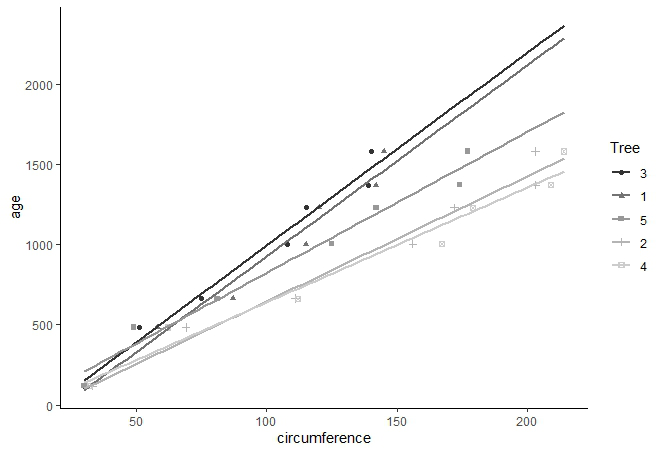My Personal Notes arrow_drop_up